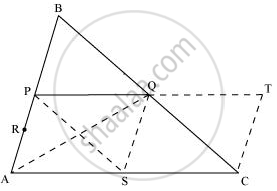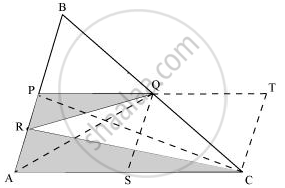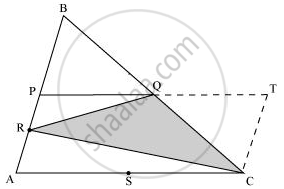Share

# P and Q are respectively the mid-points of sides AB and BC of a triangle ABC and R is the mid-point of AP, show that - CBSE Class 9 - Mathematics

ConceptTriangles on the Same Base and Between the Same Parallels

#### Question

P and Q are respectively the mid-points of sides AB and BC of a triangle ABC and R is the mid-point of AP, show that

(i) ar(PRQ) = 1/2 ar(ARC)

(ii) ar(RQC) = 3/8 ar(ABC)

(iii) ar(PBQ) = ar(ARC)

#### Solution

Take a point S on AC such that S is the mid-point of AC.

Extend PQ to T such that PQ = QT.

Join TC, QS, PS, and AQ.In ΔABC, P and Q are the mid-points of AB and BC respectively. Hence, by using mid-point theorem, we obtain

PQ || AC and PQ = 1/2AC

⇒ PQ || AS and PQ = AS (As S is the mid-point of AC)

∴ PQSA is a parallelogram. We know that diagonals of a parallelogram bisect it into equal areas of triangles.

∴ ar (ΔPAS) = ar (ΔSQP) = ar (ΔPAQ) = ar (ΔSQA)

Similarly, it can also be proved that quadrilaterals PSCQ, QSCT, and PSQB are also parallelograms and therefore,

ar (ΔPSQ) = ar (ΔCQS) (For parallelogram PSCQ)

ar (ΔQSC) = ar (ΔCTQ) (For parallelogram QSCT)

ar (ΔPSQ) = ar (ΔQBP) (For parallelogram PSQB)

Thus,

ar (ΔPAS) = ar (ΔSQP) = ar (ΔPAQ) = ar (ΔSQA) = ar (ΔQSC) = ar (ΔCTQ) = ar (ΔQBP) ... (1)

Also, ar (ΔABC) = ar (ΔPBQ) + ar (ΔPAS) + ar (ΔPQS) + ar (ΔQSC)

ar (ΔABC) = ar (ΔPBQ) + ar (ΔPBQ) + ar (ΔPBQ) + ar (ΔPBQ)

= 4 ar (ΔPBQ)

⇒ ar (ΔPBQ) = 1/4 ar(ΔABC) ... (2)

(i)Join point P to C.In ΔPAQ, QR is the median.

therefore ar(trianglePRQ)=1/2ar(trianglePAQ)=1/2xx1/4ar(triangleABC)=1/8ar(triangleABC)"          .........(3)"

In ΔABC, P and Q are the mid-points of AB and BC respectively. Hence, by using mid-point theorem, we obtain

PQ = 1/2AC

AC = 2PQ ⇒ AC = PT

Also, PQ || AC ⇒ PT || AC

Hence, PACT is a parallelogram.

ar (PACT) = ar (PACQ) + ar (ΔQTC)

= ar (PACQ) + ar (ΔPBQ [Using equation (1)]

∴ ar (PACT) = ar (ΔABC) ... (4)

ar(triangleARC) = 1/2ar(trianglePAC)"                    (CR is the median of ΔPAC)"

= 1/2xx1/2ar(PACT)"   (PC is the diagonal parallelogram PACT)"

= 1/4 ar(PACT) = 1/4 ar(triangleABC)

rArr 1/2ar(triangleARC) = 1/8ar(triangleABC)

rArr1/2ar(triangleARC)=ar(trianglePRQ)"      [Using equation (3)]    .......(5)"

(ii)ar(PACT) = ar(ΔPRQ) + ar(ΔARC) + ar(ΔQTC) + ar(ΔRQC)

Putting the values from equations (1), (2), (3), (4), and (5), we obtain

ar(ΔABC) = 1/8ar(ΔABC) + 1/4ar(ΔABC) + 1/4ar(ΔABC) + ar(ΔRQC)

ar(ΔABC) = 5/8ar(ΔABC) + ar(ΔRQC)

ar(ΔRQC) = (1-5/8)ar(ΔABC)

ar(ΔRQC) = 3/8ar(ΔABC)

(iii) In parallelogram PACT,

ar(ΔARC) = 1/2ar(ΔPAC)"           (CR is the median of ΔPAC)"

= 1/2xx1/2ar(PACT)"     (PC is the diagonal of parallelogram PACT)"

= 1/4ar(PACT)

= 1/4ar(ABC)

= ar(ΔPBQ)

Is there an error in this question or solution?

#### APPEARS IN

NCERT Solution for Mathematics Class 9 (2018 to Current)
Chapter 9: Areas of Parallelograms and Triangles
Ex. 9.40 | Q: 7 | Page no. 166

#### Video TutorialsVIEW ALL 

Solution P and Q are respectively the mid-points of sides AB and BC of a triangle ABC and R is the mid-point of AP, show that Concept: Triangles on the Same Base and Between the Same Parallels.
S